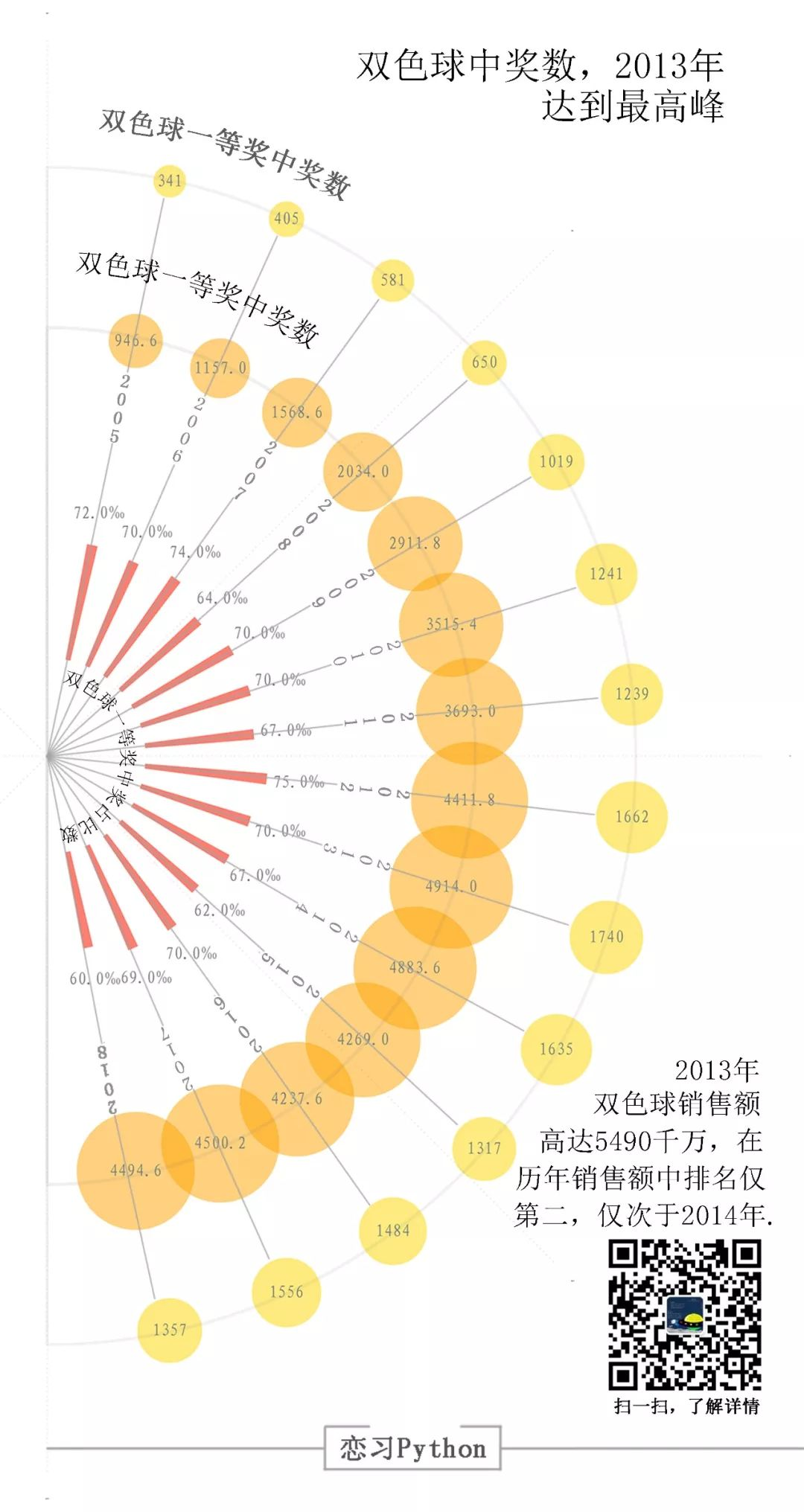# 5700億，16227注！15年來，雙色球一等獎中獎占比率幾乎不變（附Python代碼，中獎人）...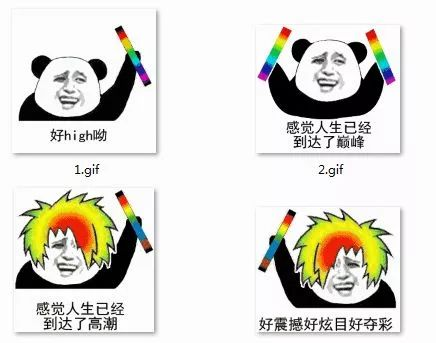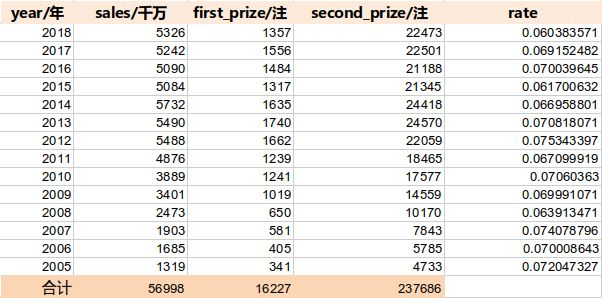2005-2018年，雙色球的數據統計

1.matplotlib.pyplot.bar(left, height, alpha=1, width=0.8, color=, edgecolor=, label=, lw=3)

left：x軸的位置序列，一般采用arange函數產生一個序列；?
height：y軸的數值序列，也就是柱形圖的高度，一般就是我們需要展示的數據；?
alpha：透明度?
width：為柱形圖的寬度，一般這是為0.8即可；?
color或facecolor：柱形圖填充的顏色；?
edgecolor：圖形邊緣顏色?
label：解釋每個圖像代表的含義?
linewidth or linewidths or lw：邊緣or線的寬度

2.matplotlib.pyplot.legend()

plt.legend()函數主要的作用就是給圖加上圖例，plt.legend([x,y,z])里面的參數使用的是list的的形式，默認情況下是將label值賦給它。

``````import?numpy?as?np
import?matplotlib.pyplot?as?plt
``````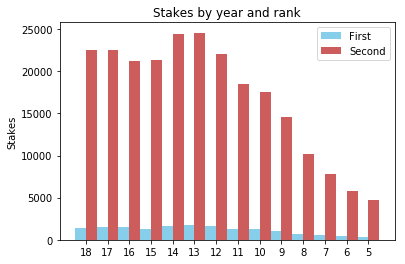• scatter(x,y)?在向量?x?和?y?指定的位置創建一個包含圓形的散點圖。該類型的圖形也稱為氣泡圖。

• scatter(x,y,sz)?指定圓大小。要繪制大小相等的圓圈，請將?sz?指定為標量。要繪制大小不等的圓，請將?sz?指定為長度等于?x?和?y?的長度的向量。

• scatter(x,y,sz,c)?指定圓顏色。要以相同的顏色繪制所有圓圈，請將?c?指定為顏色名稱或 RGB 三元數。要使用不同的顏色，請將?c?指定為向量或由 RGB 三元數組成的三列矩陣。

``````import?pandas?as?pd
import?numpy?as?np
import?seaborn?as?sns
``````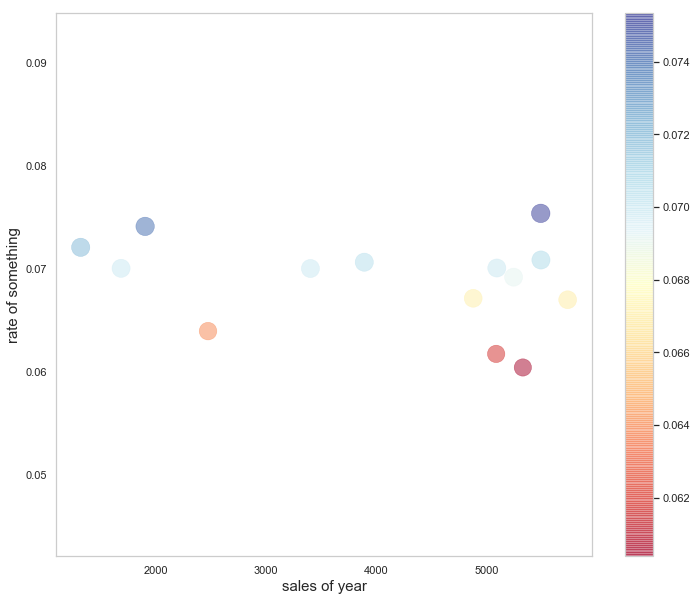、用Python畫一張好看的氣泡圖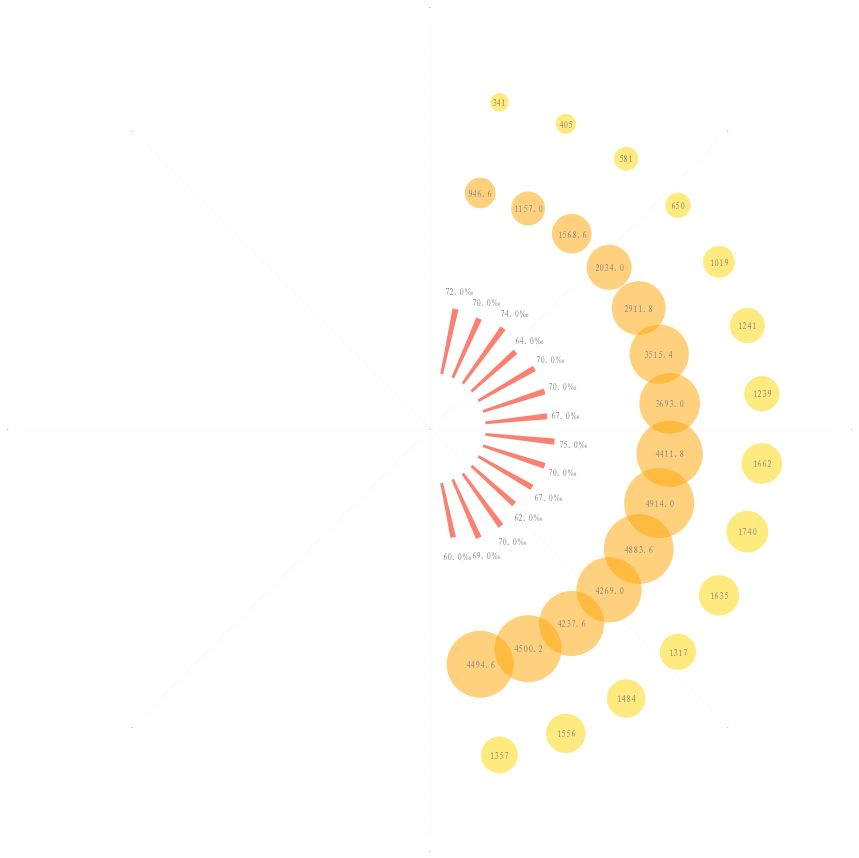``````import?numpy?as?np
import?pandas?as?pd
import?matplotlib.pyplot?as?plt
import?matplotlib.font_manager#讀取數據
first_prize?=?fp.first_prize
second_prize?=?fp.second_prize
rate?=?fp.rate#一等獎中獎注數
S1=first_prize
x1=[(3/2)*np.pi+np.pi/(len(S1)+1)*(i+1)?for?i?in?range(2*(len(S1)+1))?if?i&lt;len(S1)]
y1=[180?for?i?in?range(2*(len(S1)+1))?if?i&lt;len(S1)?]#為了畫出效果比例好看，將二等獎注數縮小5倍來表示
S2=second_prize/5
x2=[(3/2)*np.pi+np.pi/(len(S1)+1)*(i+1)?for?i?in?range(2*(len(S1)+1))?if?i&lt;len(S1)]
y2=[130?for?i?in?range(2*(len(S1)+1))?if?i&lt;len(S1)?]H1=[60/2,69/2,70/2,62/2,67/2,70/2,75/2,67/2,70/2,70/2,64/2,74/2,70/2,72/2]
W1=[0.05?for?i?in?range(2*(len(S1)+1))?if?i&lt;len(S1)]
B=[30?for?i?in?range(2*(len(S1)+1))?if?i&lt;len(S1)]fig=plt.figure(figsize=(13.44*2,7.5*2),facecolor='w')#建立一個坐標系，projection='polar'表示極坐標
ax.scatter(x=x1,y=y1,s=S1,color='Gold',alpha=0.5,linewidths=0)
ax.scatter(x=x2,y=y2,s=S2,color='Orange',alpha=0.5,linewidths=0)
ax.bar(x=x1,?height=H1,?width=W1,bottom=B,color='Salmon')plt.ylim(0,220)
#去掉坐標軸的為外面一層粗的線
ax.spines['polar'].set_visible(False)
plt.grid(color='gray',?linestyle=':',?linewidth=1,which='major',axis='x',alpha=0.1)
ax.set_yticks([])
ax.tick_params(axis='x',labelsize=0)font=matplotlib.font_manager.FontProperties(fname=r'E:\project\wordcloud\simfang.ttf')for?i?in?range(len(x1)):ax.text(x=x1[i],y=y1[i],s=S1[i],color='gray',ha='center',?va=?'center',fontsize=12,fontproperties=font)
for?i?in?range(len(x1)):ax.text(x=x1[i],y=y2[i],s=S2[i],color='gray',ha='center',?va=?'center',fontsize=12,fontproperties=font)
for?i?in?range(len(x1)):ax.text(x=x1[i],y=H1[i]+40,s="{}‰".format(H1[i]*2)?,color='gray',ha='center',?va=?'center',fontsize=12,fontproperties=font)plt.show()
fig.savefig('D:\\test.png',dpi=400,bbox_inches='tight',transparent=False)
``````